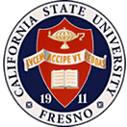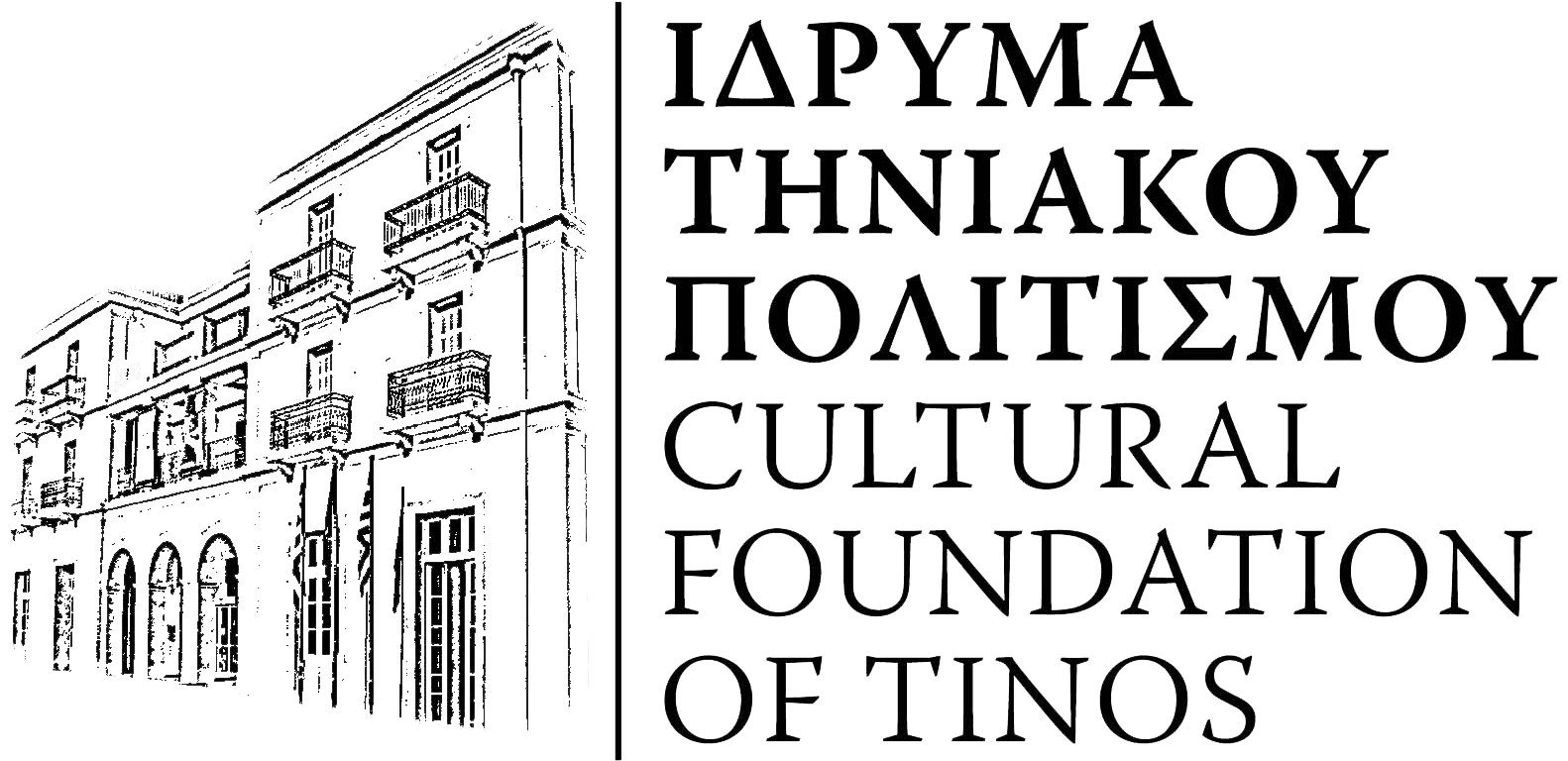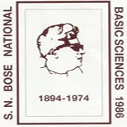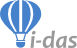# 10th International Conference on Mathematical Modeling in Physical Sciences

##### Virtual, on-line Conference### Virtual IC-MSQUARE

The virtual room will be open until the end of September. You can visit the Virtual Room by log into your account and select Program - Schedule from the main menu.

Instructions on how to create your video virtual presentation can be found here.

## Proceedings LicenceAll authors publishing in IOP Journal of Physics: Conference Series should be aware of the Licence Terms and Conditions which can be found here.

## Proceedings contents

1. Visualizing numerical solutions of linear second order differential equations with Euler's method and Lagrange interpolation via GeoGebra
Olivares Funes J. , Valero E.
2. Numerical solution of Hermite differential equations using the spline method of order 1 with GeoGebra
Olivares Funes J. , Valero E.
3. Statistical modelling of factor analysis to set causals of hybrid learning success during Covid-19 lockdown
Vazquez-Sanchez A. , Cruz Villar C. , Delgado F. , Chavez-Alcaraz E. , Chong-Quero J.
4. Post-selected double teleportation and the modelling of its related non-local properties
5. Galarkin Method with GeoGebra in Differential Equations
Olivares Funes J. , Valero E. , Martin P.
6. The Spherical Bessel function j0 in fractional differential equations
Olivares Funes J. , Martin P. , Valero E.
7. The effects of gravitational potential on chemical reaction rates
Lecca P.
8. Development of engineering calculator to copmutation the heat flux
Melekhin A.
9. On the asymptotic stability of advection-diffusion equations of mass transport in bubble column bioreactor
Lecca P. , Re A.
10. Gamma variance model: Fractional Fourier Transform (FRFT)
Nzokem A.
11. Classes of Dynamic Systems with Various Combinations of Multipliers in Their Reciprocal Polynomial Right Parts
Andreeva I.
12. Study of the stability for three-dimensional states of dynamic equilibrium of the electron Vlasov-Poisson gas
Gubarev Y. , Liu Y.
13. Stochastic Analysis of SIS Epidemic Model
Nzokem A.
Espinoza-Ortiz J. , Lagos-Monaco R.
15. Differential operators and Higher Specht polynomials
Nonkané I.
16. Differential operators and reflections groups of type \$B_n\$
Nonkané I. , Lawson L.
17. Differential operators and reflections groups of type \$D_n\$
Nonkané I. , Lawson L.
18. On the UPMSat-2 Attitude Determination and Control Subsystem’s magnetometers integration
Rodríguez E. , Cubas J.
19. FDS simulation of smoke backlayering in emergency lay-by of a road tunnel with longitudinal ventilation
Weisenpacher P. , Glasa J. , Valasek L. , Kubisova T.
20. Geometry, Coordinatization and Cardinality of the Rational Numbers from Physical Perspectives
Ghosh K.
21. Bearing friction effect on cup anemometer performance modelling
Alfonso-Corcuera D. , Pindado S. , Ogueta-Gutiérrez M. , Sanz-Andrés A.
22. On Harary energy and Reciprocal distance Laplacian energies
Trigo M.
23. Spectral radius of the Harary matrix of the join product of regular graphs
Medina L. , Trigo M.
24. An improved Trapezoidal rule for numerical integration
Abdulhameed A. , Memon Q.
25. An impedance approach to the response of matter
Vesely S. , Dolci C. , Dolci S.
26. Hilbert's 6-th problem and principle of completeness in dynamics
Tertychny-Dauri V.
27. RAD_IQ: A free software for characterization of digital X-ray imaging devices based on the novel IEC 62220-1-1:2015 International Standard
Konstantinidis A. , Martini N. , Koukou V. , Fountos G. , Kalyvas N. , Valais I. , Michail C.
28. X-ray Detection and spectral compatibility properties of Cerium Bromide (CeBr3) single crystal for medical imaging applications
Linardatos D. , Velissarakos K. , Valais I. , Fountos G. , Konstantinidis A. , Kalyvas N. , Kandarakis I. , Michail C.
29. Darboux transformations for Dirac equations with arbitrary diagonal potential matrix in two dimensions
Schulze-Halberg A.
30. The atomic structure of chemical elements in the theory of compressible oscillating ether
Magnitskii N.
31. Bounds on spectral radius of Harary matrix
Medina L.
32. On the spectral radius of the Reciprocal distance signless Laplacian matrix
Medina L.
33. Crossover from BKT-Rough to KPZ-Rough Surfaces for Crystal Growth or Recession
Akutsu N.
34. Fractal behaviors of networks induced on infinite tree structures by random walks
Ikeda N.
35. Dissociation and recombination in the electrolyte flow model
Shobukhov A. , Koibuchi H.
36. Mathematical Modeling of Soliton-Like Modes at Optical Rectification
Kalinovich A. , Zakharova I. , Sazonov S. , Komissarova M.
37. Non-smooth Modeling and Variable Structure Control of a Class of Hybrid Dynamical Systems
Bin Salamah Y.
38. A Physical Action Principle for Biological Systems
Summers R.
39. Estimation of the trajectories of magnetic nanoparticles in non-newtonian vascular fluid using neural networks
Contreras Rodríguez I. , Barragán Vargas D. , Camargo L.
40. The longtime global climatic consequences modeling of the Chicxulub asteroid impact event
Parkhomenko V.
41. Design and first simulations of the TASEC-Lab power subsystem
Marín-Coca S. , González-Bárcena D. , Pindado S. , Roibás-Millán E.
42. Analysis of chosen numerical methods for the application in high order interior ballistics simulations
Krol M.
43. The study of the dynamics for electorate system by using q-distributions-a case study.
Prenga D. , Peqini K. , Osmanaj R.
44. Energy-Variation Analysis and Orbit Complexity Quantification
Kasolis F.
45. Analytical modelling of a novel test for determination of porosity and permeability of porous materials
Torrent R. , Zino G.
46. Scaling laws and phase space analysis of a geomagnetic domino model
Peqini K. , Prenga D. , Osmanaj R.
47. Generalized IBL models for gravity-driven flow over inclined surfaces
D'alessio S. , Mastrogiacomo J. , Pascal J.
48. Determining the best set of Molecular Descriptors for a Toxicity Classification Problem
Toppur B.
49. Modeling of the content of the physics course based on the percolation coefficient
Gnitetskaya T. , Tsoy A.
50. A machine learning approach of finding the optimal anisotropic SPH kernel
Marinho E.
51. On the satellite attitude determination using simple environmental models and sensor data
Porras-Hermoso A. , Cubas J. , Pindado S.
52. Understanding the outbreak of COVID-19 in Ecuador
Tene T. , Guevara M. , Svozilík J. , Vacacela Gomez C.
53. A general method for rotational averages
Nessler R. , Begzjav T.
54. Solving partial differential equations in deformed grids by estimating local average gradients with planes
Pohjonen A.
55. Experimental Validation of the Mean Pitch Theory
Meda T. , Rogala A.
56. A Method of Determining the Parameters in systems with serialized Current-Voltage Characteristics
Ocaya R. , Yakuphanoǧlu F.
57. Nonexistence of global solutions for damped abstract wave equations with memory
Esquivel-Avila J.
58. Network analysis of nanoscale energy conversion processes
Einax M.
59. Beyond the brain : towards a Mathematical Modeling of Emotions
Ambrosio B.
60. Predictive modeling on the example of a gas turbine plant
Echkina E. , Lvov V.
61. Problems of increasing the efficiency of using fixed and working capital of petrochemical enterprises
Galeeva G.
62. EFFICIENT NUMERICAL METHOD FOR SOLVING ADVECTION-DIFFUSION-REACTION PROBLEMS ON A SPHERE
Skiba Y. , Cruz-Rodríguez R. , Filatov D.
63. Dark Matter in the Standard Model Extension in Non-commutative Geometry (NCG)
Berkane A. , Boussahel M.
64. Human machine interface (HMI) based on a multi-agent system in a water purification plant.
Mendoza Merchán E. , Andramuño Cando J. , Núñez Álvarez J. , Córdova Rivadeneira L.
65. Scaling analysis of a physics-guided kinetic energy density expansion
Aldossari B. , Al-Aswad A. , Alharbi F.
66. A general one-dimensional traffic model for motion of molecular motors on microtubules of variable length
Aldrich E. , Reed B. , Stoleriu L. , Mazilu D. , Mazilu I.
67. A one-dimensional modified TASEP model on a track of variable length: analytical and computational results
Reed B. , Aldrich E. , Stoleriu L. , Mazilu D. , Mazilu I.
68. Intelligent multi-agent architecture for a supervisor of a water treatment plant.
Mendoza Merchán E. , Andramuño Cando J. , Núñez Álvarez J. , Córdova Rivadeneira L.
69. A Generalized Pattern Search Algorithm Methodology for solving an Under-Determined System of Equality Constraints to achieve Power System Observability using Synchrophasors
Theodorakatos N. , Lytras M. , Babu R.
70. Analysis of the error distribution density convergence with its orthogonal decomposition in navigation measurements
Vorokhobin I. , Zhuravska I. , Burmaka I. , Kulakovska I.
71. The Extension of the Physical and Stochastic Problems to Space-Time-Fractional Differential Equations
Abdel-Rehim E.
72. Lower bound for the spectral radius of the starlike trees
Arrizaga-Zercovich R.
73. Upper bound for the energy of the starlike trees
Arrizaga-Zercovich R. , Medina L.
74. Quantum Vacuum Gravitation. Matter-Antimatter Antigravity.
Meis C.
75. Mathematical modelling of optical radiation transport in biological tissues under the conditions of moveable integrating spheres registration
Karpova T. , Kovalenko N. , Aloian G. , Ryabushkin O.
76. Nonlinear dynamics for the 3D ideal viscous gas flow over the cylinder
Evstigneev N. , Ryabkov O.
77. Disconnected stationary solutions for 3D Kolmogorov flow problem: preliminary results
Evstigneev N.
78. Review on de Bruijn shapes in one, two and three dimensions
Roig P. , Alcaraz S. , Gilly K. , Bernad C. , Juiz C.
79. Supercomputer power consumption predictions using machine learning, nonlinear algorithms, and statistical methods
Tomčala J.
80. The correlation analysis of the daily Covid-19 new cases data series in Albania.
Gjana A. , Kovaçi S.
81. Secondary Faraday waves in microgravity
Labrador Vares E. , Salgado Sánchez P. , Porter J. , Shevtsova V.
82. High-speed camera test of newly developed igniter's charges for artillery munition
Plachá A. , Recko J.
83. The influence of knot types on rope static tensile strength
Rybakiewicz . , Plachá A. , Ruliński P.
84. Performance Characterization of Dual-Metal Triple-gate-dielectric (DM_TGD) Tunnel Field Effect Transistor (TFET)
Chun Fui T. , Singh A. , Way Soong L.
85. The influence of accidental physical contacts between individuals on viral infection
Derevich I. , Panova A.
86. Boundary value problems for a higher order Sobolev equation of mixed type
Fedorov V.
87. Synthesis of a time-optimal control system for an extremal object
Pikina G. , Pashchenko F. , Pashchenko A.
88. Geometrical model of diffuse reflection of optical radiation from the surface of biological tissues implemented on the basis of two-dimensional "fractional Brownian motion" process
Smirnov A. , Kovalenko N. , Ryabushkin O.
89. Numerical simulation of changes in the electrical properties of biological tissues under local heating by laser radiation
Kovalenko N. , Smirnov A. , Ryabushkin O.
90. Numerical solution of generalized fractional Volterra integro-differential equations via approximation the Bromwich integral
Eshaghi S. , Ordokhani Y.
91. Mathematical modelling of fluid flow through porous media with heterogeneity of permeability
Astafev V.
92. Implementing the basic CT backprojecting algorithm in android mobile application
Kussainov A. , Em M. , Myrzabek Y. , Mukhatay M.
93. Derivation of the Symmetric Stress-Energy-Momentum Tensor in Exterior Algebra
Colombaro I. , Font-Segura J. , Martinez A.
94. CNF-SAT Modeling for banyan-type networks and its application for assessing the rearrangeability
Ohta S.
95. Performances of different DFT functionals to calculate the anodic limit of fluorinated sulphonyl-imide anions for lithium cells
Paolone A. , Brutti S.
96. On Symmetric Solutions to Linear Matrix Time-Varying Differential Equations
Fetisov D.
97. Model of collisions between magnetic nanoparticles and the arterial wall
Garzón Suárez D. , Camargo L. , Rodríguez Patarroyo D.
98. Thermodynamic analysis of oligomeric blends applying Kirkwood-Buff theory of solutions
Venetsanos F. , Anogiannakis S. , Theodorou D.
99. Determination of ship roll damping coefficients by a differential evolution algorithm
Mauro F. , Nabergoj R.
100. Molecular Dynamics Simulations of Polyethylene Bilayers in the Melt State
Romanos N. , Megariotis G. , Theodorou D.
101. Generation of new symmetries from an explicit symmetry breaking
Dimakis N.
102. A higher-order numerical analysis to study the flow physics and to optimize the design of a short-dwell blade coaters for higher efficiency
Sahoo B. , Mahato B. , Sekhar T.
103. OPTIMAL EMISSION CONTROL AND IDENTIFICATION OF AN UNKNOWN POLLUTION SOURCE
Parra-Guevara D. , Skiba Y.
104. Gravity assists gravitational scattering and the perturbation rings in the Solar system
Grushevskii A.
105. Accelerating the charge inversion algorithm with hierarchical matrices for gas insulated systems
Lucchini F. , Marconato N.
106. A comparison between current-based integral equations approaches for eddy current problems
Lucchini F. , Marconato N.
107. Rutherford's extended formula for the gravitational scattering and the synthesis of gravity assists chains in the Solar system
Grushevskii A.
108. Thermodynamic Analysis of the Relaxation Model for Non-Equilibrium Phase Behavior of Hydrocarbon Mixtures
Indrupskiy I. , Chageeva P.
109. Adjoint Numerical Method for a Multiphysical Inverse Problem of Two-Phase Well Testing in Petroleum Reservoirs
Shishkina O. , Indrupskiy I.
110. One-dimensional thermal equation modeled on a two-dimensional heat exchanger with variable solid-fluid interface
Ishida H. , Higuchi K. , Hirahata T.
111. Frequency analysis in an infinite beams array
Baquero H.
112. Semi-Analytical Method for Accurate Calculation of Well Injectivity During Hot Water Injection for Heavy Oil Recovery
Indrupskiy I. , Bukatkina A.
113. Application of the form invariance transformations of the scalar cosmological model in inflation theory
Razina O. , Tsyba P. , Suikimbayeva N.
114. Parameter estimation for a simplified model of an electrolytic capacitor in transient regimes
Barbulescu C. , Dragomir T.
115. Monte Carlo studies on shape deformation and stability of 3D skyrmions under mechanical stresses
Koibuchi H. , Hongo S. , Kato F. , Diguet G. , Uchimoto T. , Hog S. , Diep H.
116. Mean-field parameters of some \$Pr_{x}Tb_{(1-x)}Al_{2}\$ compounds found via searching for the best magnetic heat capacity fitting
Tedesco J. , Monteiro V. , De Carvalho A. , Cardoso L. , Coelho A.
117. Attempt to quantify the impact of seasonal air density variation on operating tip-speed ratio of small wind turbines
Suzuki H. , Hasegawa Y. , Afolabi O. , Mochizuki S.
118. Impact of difference between explicit and implicit second-order time integration schemes on isotropic/anisotropic steady incompressible turbulence field
Honda R. , Suzuki H. , Mochizuki S.
119. A layer potential approach to functional and clinical brain imaging
Asensio P. , Badier J. , Leblond J. , Marmorat J. , Nemaire M.
120. On Multiquadric Shape Determining Strategies in Image Reconstruction Applications: A Comparative Study
Kaennakham S. , Sriapai N. , Paewpolsong P. , Ritthison D.
121. Group classification of the generalized Klein-Gordon equation by the method of indeterminates
Ndogmo J.
122. Structural health monitoring of tall buildings: the case of Enel Skyscrape
Frunzio G. , Di Gennaro L.
123. Out‑of‑plane behaviour of masonry infills
Frunzio G. , Di Gennaro L.
124. Weather in field artillery ballistics calculation
Mendel M.
125. Solving bio-heat transfer multi-layer equation using Green's Functions method
Peixoto De Oliveira E. , Guimarães G.
126. Quantized toroidal dipole eigenvalues in nano-systems
Preda A. , Anghel D.
127. Power law distributions in a new toy model with interacting \$N\$ agents
Tashiro T.
128. Photoacoustic spectroscopy allows to make correlations between blood p450 cytochrome and glycemia type 1 experimental diabetes
Olvera Cano L. , Villanueva López G. , Romero Mateos E. , Cruz Orea A.
129. Prediction of the microstructural grain evolution during selective laser melting by a cellular automata method
Wang L.
130. General properties of exchange rate of national money versus some foreign currencies in Albania
Gjana A. , Kovaçi S.
131. On the distribution of the atomic planes in an elastic single-crystal bar under the action of volumetric forces
Karyev L. , Fedorov V. , Berezner A.
132. THE INFLUENCE OF JOINT GEOMETRY OF TONEHOLES WITH THE MAIN BORE OF WIND MUSICAL INSTRUMENTS ON THEIR FREQUENCY CHARACTERISTICS
Gerasimov R.
133. Noether symmetry approach in \$f(T,B)\$ teleparallel gravity with a fermionic field
Myrzakulov K. , Myrzakulov Y. , Bekov S.
134. Does the Zero Carry Essential Information for Artificial Neural Network learning to simulate the contaminant transport in Urban Areas?
Berendt-Marchel M. , Wawrzynczak A.
135. Hybrid modeling of the human cardiovascular system using NeuralFMUs
Thummerer T. , Tintenherr J. , Mikelsons L.
136. Soliton solutions of the cubic-quintic complex Ginzburg-Landau equation in the presence of higher-order effects
Ferreira M.
137. Group velocity dispersion in terahertz frequency combs within a generalized Maxwell-Bloch framework
Seitner L. , Popp J. , Riesch M. , Haider M. , Jirauschek C.
138. Advanced selection of ensemble control tools
Samoletov A. , Vasiev B.
139. A benchmark study on the model-based estimation of the go-kart sideslip angle
Arricale V. , D'inverno M. , Zanardi A. , Frazzoli E. , Sakhnevych A. , Timpone F.
140. Modelling a mechanical antenna for a calibrator for interferometric gravitational wave detector using finite elements method
Prado A. , Bortoli F. , Magalhaes N. , Duarte R. , Frajuca C. , Souza R.
141. Obtaining the sensitivity of a calibrator for interferometric gravitational wave
Prado A. , Bortoli F. , Magalhaes N. , Duarte R. , Frajuca C. , Souza R.
142. Monitoring the brightness temperature of the Moon throughout the lunar cycle from radio observations in the Ku band
Galeano D. , Quintero E.
143. Modelling a suspension for an experiment to measure the speed of gravity in short distances
Ramalho W. , Bortoli F. , Magalhaes N. , Duarte R. , Frajuca C. , Souza R.
144. Obtaining the frequencies of Schenberg detector sphere using finite element modelling
Bortoli F. , Duarte R. , Souza R. , Magalhaes N. , Frajuca C. , Souza S.
145. Optimum Placement of Long Gauge FBG Sensor in Reinforced Concrete Bridge: A Case Study
Lala S. , Basumallick N. , Biswas P. , Bandyopadhyay S.
146. RESONANCES OF ELECTROMAGNETIC-INDUCED TRANSPARENCY AND ELECTROMAGNETIC-INDUCED ABSORPTION AT THE TRANSITION WITH LEVEL MOMENTA J=1/2 IN UNIDIRECTIONAL WAVE SPECTROSCOPY
Chernenko A. , Saprykin E.
147. Identification test of elements remaining in the barrel after firing, using Differential Scanning Calorimetry
Plachá A. , Prasuła P. , Recko J.
148. Grid-characteristic numerical method for medical ultrasound
Beklemysheva K. , Vasyukov A. , Ermakov A.
149. Nonlocal Schro ̈dinger-Maxwell-Bloch Equations
Umurzakhova Z. , Yesmakhanova K. , Naizagarayeva A. , Meirambek U.
150. Features of the multi-criteria optimization mathematical model of the thermal and electrical loads distribution at a combined heat and power plant with a mixed equipment composition
Arakelyan E. , Andryushin A. , Mezin S. , Kosoy A.
151. On the position dependent mass Schrödinger equation for Mie-type potentials
Ovando G. , Peña J. , Morales J. , López-Bonilla J.
152. Methodological Bases of Optimal Load Distribution at a Thermal Power Plant with a Complex Composition of Equipment, Taking into Account Market Requirements
Arakelyan E. , Andryushin A. , Yagupova Y. , Neklyudov A. , Mezin S.
153. Photosynthetic pigment-protein complexes optical response modeling optimized by Differential evolution: algorithm convergence study
Chesalin D. , Pishchalnikov R.
154. PGU-450 network heaters modes modelling with CCGT participation in regulating the electrical load in the heating mode
Arakelyan E. , Andryushin A. , Mezin S. , Yagupova Y.
155. Seeing the unseen–the iceberg phenomenon in the first months of the COVID19 pandemic
Anghel D. , Anghel I.
156. Statistical approaches to the problem of homogeneous melting of solids in the microcanonical ensemble
Olguín-Arias V. , Davis S. , Gutiérrez G.
157. The theory of the chain fountain revisited
Anghel D.
158. Travelling wave solutions for the generalized nonlinear Schro ̈dinger equation
Shaikhova G. , Rakhimzhanov B. , Zhanbosinova Z.
159. Third party stabilization of unstable coordination in systems of coupled oscillators
Mckinley J. , Zhang M. , Wead A. , Williams C. , Tognoli E. , Beetle C.
160. Variational approach to dispersion-mamaged solitons in polarization preserving and birefringent fibers
Ferreira M.
161. Generalized F(R,T) cosmological models with fermionic fields
Yerzhanov K. , Bauyrzhan G. , Myrzakulov R.
162. Weak correlation between fluctuations in protein diffusion inside bacteria
Itto Y. , Beck C.
163. Assessment and ranking flood events in a regulated river using information and complexity measures
Al Sawaf M.
164. Internal Geneva Mechanism Geometric Resolution
Martinez Escobedo E.
165. Deconvolution of induced spatial discretization filters subgrid modeling in LES: application to two-dimensional turbulence
Boguslawski A. , Wawrzak K. , Paluszewska A. , Geurts B.
166. Mathematical Model Considering Effect of COVID-19 Contact-Confirming Application (COCOA) and “GoTo Travel Campaign”
Maehashi R. , Nagaoka R. , Nigoshi Y. , Hayashi Y. , Moriguchi R. , Kakimoto Y. , Toyotani J. , Hara K. , Takahashi H. , Omae Y.
167. The Effect of Privacy Concerns, Risk, Control and Trust on Individuals' Decisions to Share Personal Information: A Game Theory-Based Approach
Dimodugno M. , Hallman S. , Plaisent M. , Bernard P.
168. Digital twin of the management process of field service teams of an electric grid company
Kinzhalieva A. , Protalinskiy O. , Khanova A. , Bondareva I.
169. Ensemble kalman filter and particle filter-based state estimation on electrical power systems
Cevallos Ulloa H. , Intriago G. , Plaza D.
170. Spatial Resolution Enhancement of Rotational-Radar Subsurface Datasets Using Combined Processing Method
Mc Donald T. , Robinson M. , Tian G.
171. Identification and Forecast of Thermal and Moisture Regime in Case of Ground Ice in Base of Gas Pipeline
Permyakov P. , Vinokurova T. , Popov G.
172. On stability boundary of the flow of an acid solution through a chemically active porous medium
Plavnik R. , Zavialov I. , Konyukhov A. , Izvekov O. , Negodyaev S.
173. Possibilities of R programming language in simulating microbiological synthesis processes
Nikitina M. , Chernukha I.
174. Modelling the water droplet motion on a leaf surface\jpcs
Oqielat M.
175. Handling Massive Proportion of Missing Labels in Multivariate Long-Term Time Series Forecasting
Freitas Iglesias Jr C. , Mehta V. , Venereo-Sanchez A. , Xu X. , Robitaille J. , Voyer R. , Richard . , Belacel N. , Kamen A. , Bolic M.
176. Evaluation of the vacuum infusion process objectives at the early stages of computer simulation
Huang J. , Zhilyaev I. , Snezhina N. , Shevtsov S.
177. Mathematical modeling of a new way of renal artery denervation
Makarov V.
178. Parallel Quantum Computation Approach for Quantum Deep Learning and Classical-Quantum Models
Payares E. , Martinez-Santos J.
179. Development of a system SIEMNED for integrated modeling of processes in tokamak installations
Zhilkin A. , Sychugov D. , Vysotsky L. , Sadykov A. , Soloviev S.
180. The (2+1) dimensional metric \$f(R)\$ gravity non-minimally coupled with fermion field
Myrzakulov N. , Tursumbayeva G. , Myrzakulova S.
181. Impact of ballistic parameters of used ammunition on stress distribution on the parts of the short recoil operated weapon
Badurowicz P. , Wróblewski A. , Kupidura P. , Fikus B.
182. Validation of a spatial-time concentration gradients estimation by the superposition of sphere sources diffusion fields using the finite element method
Nartsissov Y.
183. Energy analysis of Duffing oscillators with quadratic damping: exact solutions
Rakaric Z. , Kovacic I.
184. On deformations of the Galilei algebra
Alvarez M. , Rosales-Gómez J.
185. Examples of S-expansions of Lie algebras
Rosales-Gómez J.
186. A novel algorithm of the digital nervous tissue phantom creation based on 3D Voronoi diagram application
Nartsissov Y.
187. Numerical Modeling of Climate (on Yakutia example)
Pomortseva A.
188. Geometric Flows of Curves, Two-Component Camassa-Holm Equation and Generalized Heisenberg Ferromagnet Equation
Nugmanova G. , Tayshieva A. , Myrzakulov R. , Myrzakul T.
189. Dipole Polarizability of C\$_{28}\$ and its Counterparts Nb\$_4\$B\$_{18}\$ and Ta\$_4\$B\$_{18}\$. Insights from a Density Functional Theory (DFT) Endeavour
Xenides D. , Karamanis P.secretariat@icmsquare.net
(+30)6944 371 526